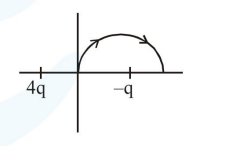# Solve this following

Question:

A two point charges $4 \mathrm{q}$ and $-\mathrm{q}$ are fixed on the

$x$-axis at $x=-\frac{d}{2}$ and $x=\frac{d}{2}$, respectively. If

a third point charge ' $q$ ' is taken from the origin

to $x=d$ along the semicircle as shown in the

figure, the energy of the charge will:1. increase by $\frac{2 q^{2}}{3 \pi \varepsilon_{0} d}$

2. increase by $\frac{3 q^{2}}{4 \pi \varepsilon_{0} d}$

3. decrease by $\frac{4 \mathrm{q}^{2}}{3 \pi \varepsilon_{0} \mathrm{~d}}$

4. decrease by $\frac{\mathrm{q}^{2}}{4 \pi \varepsilon_{0} \mathrm{~d}}$

Correct Option: , 3

Solution:

Potential of $-\mathrm{q}$ is same as initial and final point of the path therefore potential due to $4 \mathrm{q}$ will only change and as potential is decreasing the energy will decrease

Decrease in potential energy $=\mathrm{q}\left(\mathrm{V}_{\mathrm{i}}-\mathrm{V}_{\mathrm{f}}\right)$

Decrease in potential energy

$=\mathrm{q}\left[\frac{\mathrm{k} 4 \mathrm{q}}{\mathrm{d} / 2}-\frac{\mathrm{k} 4 \mathrm{q}}{3 \mathrm{~d} / 2}\right]=\frac{4 \mathrm{q}^{2}}{3 \pi \varepsilon_{0} \mathrm{~d}}$

Therefore correct answer is 3 .